# Civil Engineering - UPSC Civil Service Exam Questions

41.

For an activity 201-207 in an AOA network, the EET values, LET values and activity duration are shown in the figure. Between free float, interference float and independent float, which one of the following is the correct sequence in the decreasing magnitude order ?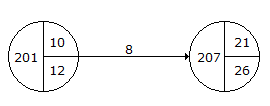A. Free float - Interference float - Independent float B. Free float - Independent float - Interference float C. Interference float - Free float - Independent float D. Interference float - Independent float - Free float

Explanation:

No answer description available for this question. Let us discuss.

42.

Consider the following statements :
2. Dry dock is generally used only for carrying out repairs, inspections and painting.
3. Wet dock is an enclosed or partially enclosed basin provided with locks and entrance gate to keep the water level at a fairly constant level.
Which of the statements given above are correct ?

 A. 1, 2 and 3 B. 2 and 3 C. 1 and 2 D. 1 and 3

Explanation:

No answer description available for this question. Let us discuss.

43.

Which one of the following statements is true to trickling filter sludge ?

 A. It has a comparatively low sludge volume index B. It is more difficult to dewater than activated sludge C. It has a comparatively low concentration of sludge solids D. It is bulky

Explanation:

No answer description available for this question. Let us discuss.

44.

In the case of a column of length 'l', moment of inertia of cross-section 'I' and Young's modulus of the material of the column 'E' being hinged at both ends, the buckling load, according to Euler's colum

 A.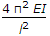B.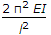C.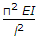D.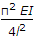Explanation:

No answer description available for this question. Let us discuss.

45.

What is the correct sequence of the following steps in the graphical determination of stresses in the members of a loaded plane truss ?
1. Vector diagram to determine the end reactions.
2. Space diagram.
3. Stress diagram.
Select the correct answer using the codes given below :

 A. 1, 2, 3 B. 1, 3, 2 C. 2, 1, 3 D. 2, 3, 1